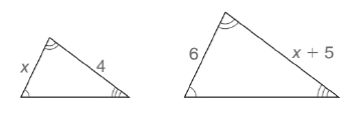# Determine the length x by solving either the equation x 4 = 6 x + 5 or the equivalent equation x x + 5 = 24 .### Elementary Geometry For College St...

7th Edition
Alexander + 2 others
Publisher: Cengage,
ISBN: 9781337614085### Elementary Geometry For College St...

7th Edition
Alexander + 2 others
Publisher: Cengage,
ISBN: 9781337614085

#### Solutions

Chapter
Section
Chapter A.4, Problem 38E
Textbook Problem

## Determine the length x by solving either the equation x 4 = 6 x + 5 or the equivalent equation x x + 5 = 24 .Expert Solution
To determine

To find:

The length ‘x’ by solving the equation xx+5=24.

### Explanation of Solution

Solving an equation is to find the value of the unknown variables in the equation, such that the obtained value or values of the unknown should satisfy the equation from which it was derived. Such a value is said to be the solution for the equation. In general a quadratic equation has two solutions for the variable in the equation as the degree of the equation is two.

Calculation:

Given,

xx+5=24

Apply the distributive property of multiplication over addition.

x·x+x·5=24

x2+5x=24

x2+5x+-24=24+-24

x2+5x-24=0

The above equation is in the standard form of a quadratic equation.

Now, we shall proceed with the factorization of the quadratic expression in the left of the equation.

First look for the common factor GCF of the terms x2, 5x and -24.

But, here the GCF is 1.

Thus, we have to factor the above equation by the reverse FOIL method.

First factorize the product of the coefficient of the first tern and the constant, such that the sum of the factors gives the coefficient of the middle term.

Thus, factorize -24 as -3·8 so that the sum of the factors (-3)+8 gives 5.

Now, split the middle term 5x as (-3x)+8x.

Hence, the equation becomes,

x2+-3x+8x-24=0

x·x+-3·x+8·x+8·-3=0

Factor out the common factors form the first two terms and form the last two terms of the above expression

### Want to see the full answer?

Check out a sample textbook solution.See solution

### Want to see this answer and more?

Bartleby provides explanations to thousands of textbook problems written by our experts, many with advanced degrees!

See solution